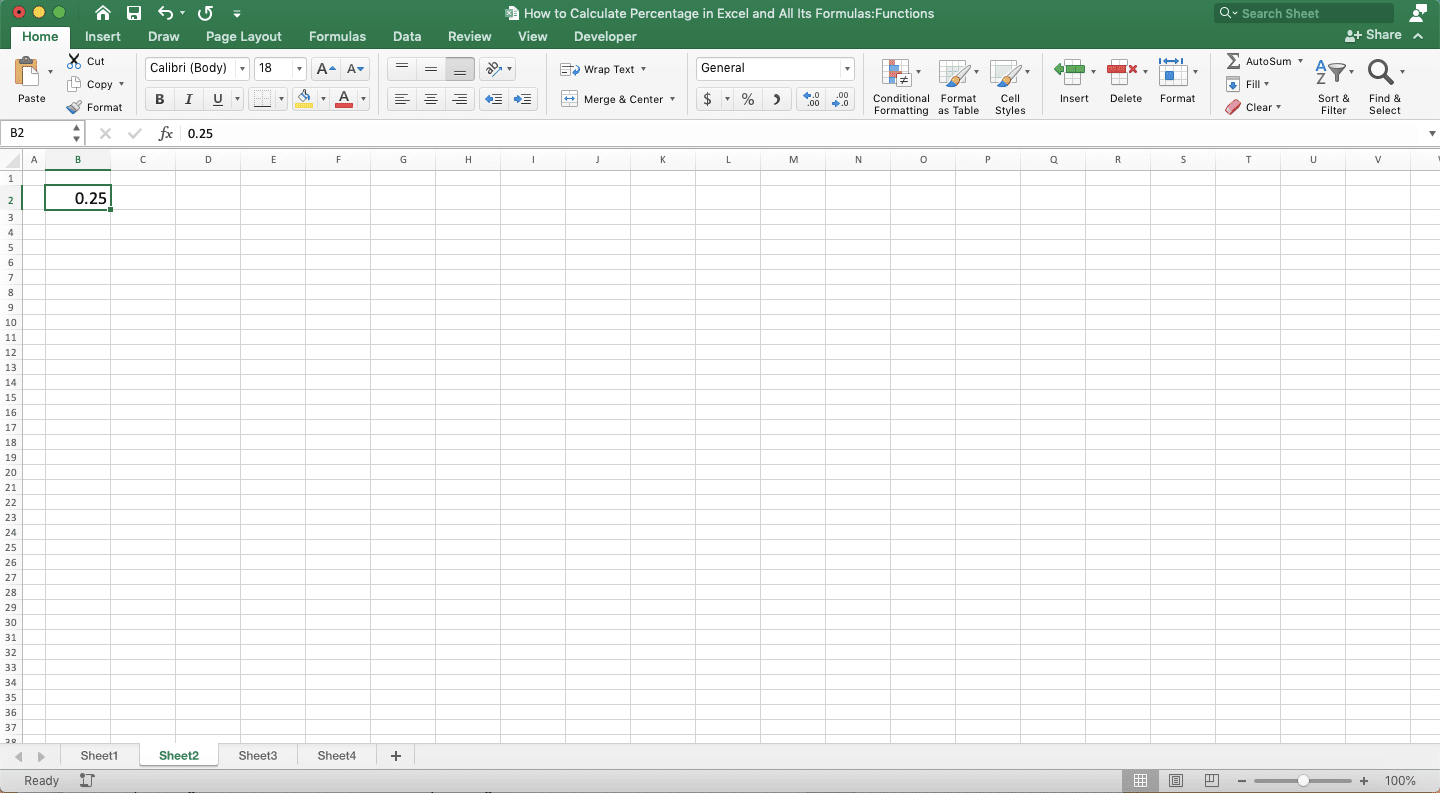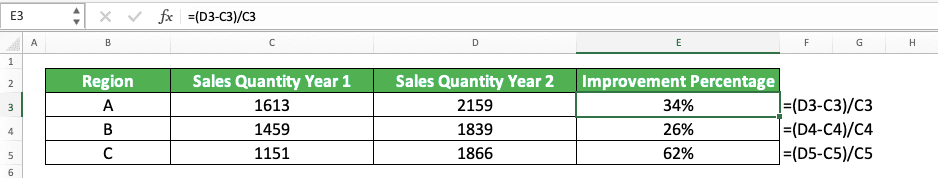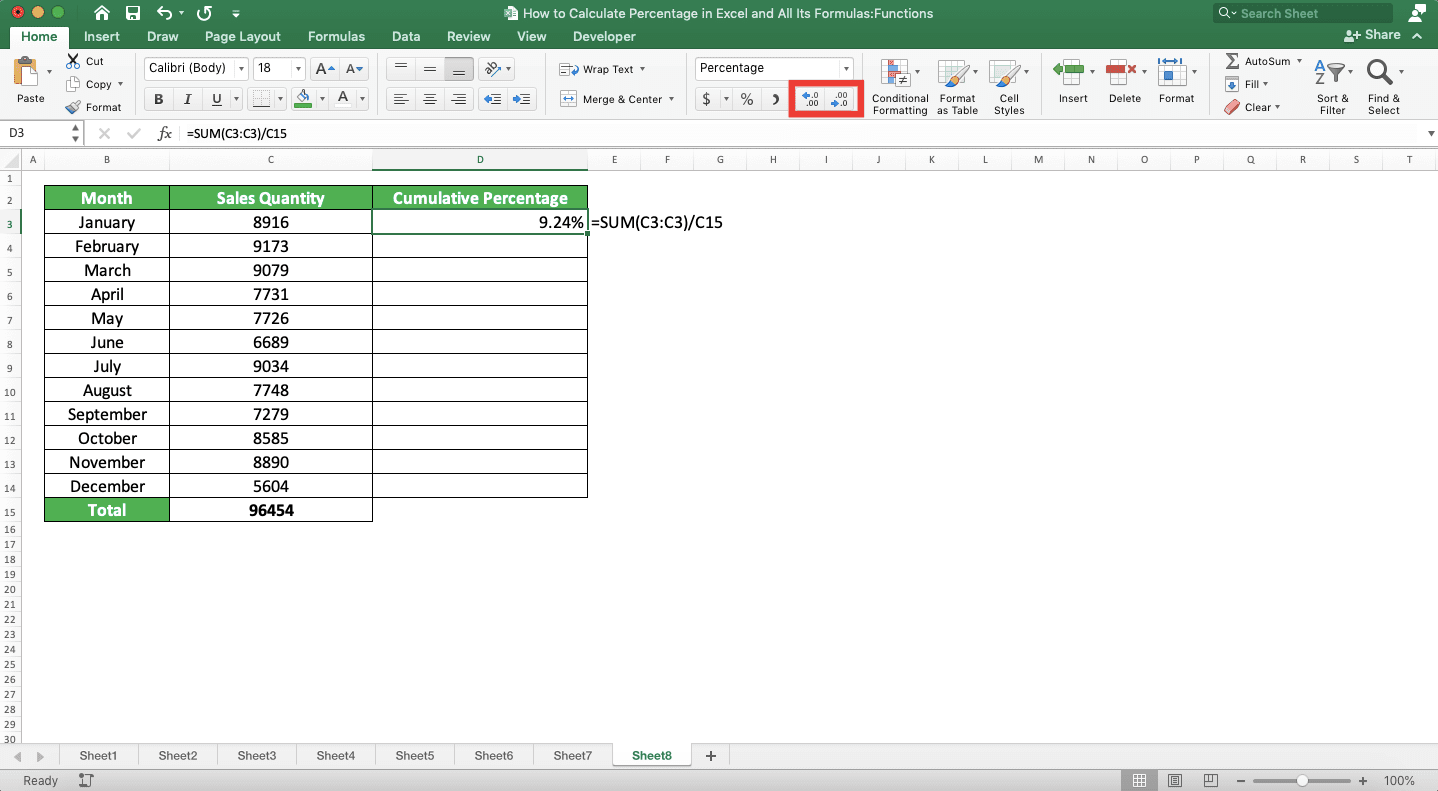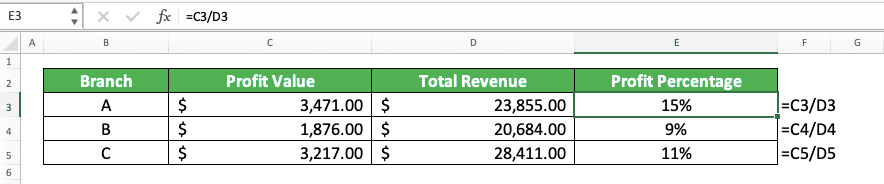How to Calculate Percentages in Excel and All Its Formulas/Functions - Compute Expert

# How to Calculate Percentages in Excel and All Its Formulas/Functions

Home >> Excel Tutorials from Compute Expert >> Excel Calculations >> How to Calculate Percentages in Excel and All Its Formulas/Functions

In this tutorial, you will learn how to calculate percentages in excel with all its formulas/functions.

When we process our numbers, a percentage calculation is one of the things we sometimes do. If we often do our numbers processing in excel, then we should understand how to calculate percentages in the software. That is if you want to get an optimal result from your excel work.

Disclaimer: This post may contain affiliate links from which we earn commission from qualifying purchases/actions at no additional cost for you. Learn more

## How to Format a Number to Percentage in Excel

The first thing we will discuss in this tutorial is the way to turn a number into a percentage in excel. This is an easy thing to do as you can see in the following explanation.

Just type a percentage symbol ( % ) behind the number that you want to convert. This will immediately make excel recognize the number as a percentage!

## How to Convert a Decimal to Percent in Excel

To immediately convert a decimal into its equivalent percent value in one go, do this.

First, select the cell where the decimal you want to convert is. Then, click the Percent Style button in the Home tab of your excel file. Your decimal will immediately change into its percent number equivalent!Alternatively, you can also convert the decimal through the format cells menu. Just choose “percentage” for the data format you want for your decimal there.

## How to Convert a Percent to Decimal in Excel

To convert a percent to decimal directly in excel, do the opposite of the method we discussed earlier.

Select the cell where the percentage you want to convert is. Then, change the data format by clicking its dropdown button in the Home tab in your excel file. Choose the General or Number data format from the dropdown list.Excel will immediately convert your percentage into its decimal equivalent!## The Basic Formula to Calculate Percentages in Excel

If you want to know the percentage of a number relative to another number in excel, you can write this formula.

= number_for_the_percentage / number_to_compare

After you get the formula result, convert it to a percentage by using the Percent Style button we have discussed before.

You can see the implementation example of the formula writing below.As you can see there, we divide the number we want to turn to a percentage with another number. As the result from this is still a number, we click the Percent Style button to turn it to percentage.

Do that and you will get your percentage calculation result!

## Formula to Calculate Proportion Percentage in Excel

To get a proportion percentage, just follow the basic percentage formula writing pattern we discussed just now. Here, you need to divide the number you want to see the proportion percentage of with the total of your numbers.

Generally, here is the way to write the formula.

= number_to_see_the_proportion_percentage_of / total

Don’t forget to click the Percent Style button on the number once you get the formula result.

## Formula to Calculate Percentage Increase in Excel

The formula writing pattern to calculate a percentage increase in excel is also similar. Here is the general formula writing form.

= increment / number_before_the_increase

If you have the number after the increase instead (not the increment itself), subtract it with the number before the increase to get the increment. Don’t forget to click the Percent Style button after you get the division result.

Here is an example of the calculation implementation in excel.In the example, we have sales quantities data in year 1 and 2. To calculate the sales quantity improvement percentage annually, we subtract year 2 sales quantity with year 1. After that, we divide the subtraction result with the year 1 sales quantity.

We can do all that in one formula writing as you can see in the example. From the calculation, we get the percentage increase results we need!

## Formula to Calculate Percentage Decrease in Excel

The way to calculate percentage decrease in excel is almost similar to the way we calculate percentage increase.

= decrement / number_before_the_decrease

However, if you need to subtract before you get the decrement, pay attention to this. If you want the percentage result to be positive, then you need to switch your numbers’ subtraction position.

Subtract the number before decrease with the number after decrease to get the decrement. Only after that you divide with the number before the decrease. Don’t forget to click the Percent Style button on your number after that.

Here is an example of the calculation implementation in excel.In the example, we have inventory numbers for day 1 and 2. If we want to calculate the decrement percentage from day 1 to day 2, we subtract the inventory numbers first.

After we get the subtraction result, we divide it with the day 1 inventory number. Don’t forget to convert the result into a percentage by using the Percent Style button.

## Growth Percentage Formula in Excel

Calculating growth is quite the same as calculating a percentage increase we have discussed before. We need to divide the growth by the number before the growth happens. Don’t forget to use the Percent Style button on the result after that.

= growth / number_before_growth

Here is the implementation example of the formula writing in excel for easier understanding.In the example, we have the number of employees data in some companies for year 1 and 2. As we want to know the growth percentage, we subtract the numbers of employees to get the growth number. From there, we divide it by the number of employees year 1 and convert the result to a percentage.

## Formula to Calculate Percent Discount in Excel

Similar to growth percentage and percent increase, the way to calculate discount percentage is similar to the percent decrease. Just divide the discount value with the price before the discount. Don’t forget to convert the division result to a percentage using the Percent Style button.

= discount_value / price_before_discount

Here is the implementation example of the calculation concept.We have the price before and after the discount and we want to know the discount percentage. Therefore, we subtract the price before discount with the price after discount. After that, we divide the result with the price before the discount.

As always, we implement the Percent Style button on the division result after that to get our percentage.

## Cumulative Percentage Formula in Excel

If you want to get cumulative percentages in excel, then you need to know your numbers total first. Place those numbers in a cell sequence and use SUM and the excel formula copy process to do the calculation easier.

To better understand the concept, see the following example. Let’s say we have a sales quantities data set like below.We want to calculate cumulative percentages of each month from January to December. For that, we have summed all the sales quantities in a total row as you can see above.

To start, we write a formula to calculate the percentage of January sales quantity compared to the total. In the formula writing, we use SUM when we input the number to divide.

We input a cell range that contains the January sales quantity cell coordinate only to the SUM. We write this SUM so we can copy this formula writing later to get all the cumulative percentages we need.

Don’t forget to also convert the formula result into a percentage format. If you want to increase/decrease the number of decimals in the percentage, use the increase decimal/decrease decimal button.Here comes the important part of the calculation of the cumulative percentages. If you want to do it fast, then add dollar symbols (\$) in the formula you just write. Add them in front of the total and SUM first cell column letter and row number references like this.You can type them yourself or press the F4 button (Command + T for Mac) when your cell cursor is in the total cell coordinate.

For those who don’t know, the \$ symbols will make a cell coordinate reference becomes an absolute reference. That means when we copy the formula anywhere, the total and SUM first cell coordinate references won’t move.

This is great for our cumulative percentages calculation as we need to always refer to them. For the SUM, as we calculate cumulative percentages, we need to sum numbers as we go with our calculation. The SUM first cell coordinate shouldn’t move because we need to sum our numbers from the start (from January sales quantity).

As for the total number, we need to refer to it all the time as the divisor in our formula. By using the \$ symbols, we can write just one formula and copy the formula to get our results!

In the example, that means we just write the cumulative percentage formula for January sales quantity. After we get the result, we copy down the formula until the December sales quantity row like this.That makes us get the cumulative percentages much faster!

## Formula to Calculate Profit Percentage in Excel

The principle of profit percentage calculation in excel is quite similar to the calculations we have discussed here previously. As a profit percentage is a comparison between profit and total revenue, here is the formula writing to get it.

= profit / total_revenue

As ever, apply the percentage data format to the result of the division. Use the Percent Style button in the Home tab to do that easily.

Here is the implementation example of the formula writing above.If you have the net revenue and not the profit, then you need to do this first. Subtract the gross (total) revenue with the net revenue first to get the profit. Then, divide it with the gross revenue as we have discussed. Don’t forget to use the percentage data format so your result will turn into a percentage.

## Formula to Calculate the Percentage of Total in Excel

To calculate the percentage of the total, you need to divide your number by the total. Therefore, the formula writing in excel becomes like this.

= number_to_know_the_percentage_of / total

Here is the implementation example of the formula writing.Just divide your number with the numbers total and use the Percent Style button on the result. You will get your percentage of the total immediately!

## Formula to Add Percentages in Excel

You can add percentages in excel just like you add numbers. Use the plus symbol ( + ) or the SUM formula to add them. If the ones you add are percentages, then the result will automatically be in a percentage form too.

Here are the writing form to add percentages in excel using the plus symbol.

= percentage1 + percentage2 …

And here is the writing form using SUM.

= SUM ( percentage1 , percentage2 , … )

As in the SUM for numbers, you can input your percentages using direct typing, cell coordinates, or cell ranges. If you give more than one inputs, then use commas ( , ) to separate them.

Here is the implementation examples of the formulas to add percentages in excel.In these examples, we want to add the percentages we have in each row. You can see how we add them using the plus symbol and SUM and get the same results.

Choose the method you prefer to add the percentages in your excel worksheets!

## Formula to Subtract Percentages in Excel

Similar to adding percentages, you should subtract percentages in excel as if you subtract numbers. That means you should also use a minus sign ( - ) in your formula writing. The subtraction result will become a percentage if the ones you subtract are all percentages.

Here is the general formula writing form of percentages subtraction in excel.

= percentage_to_subtract - percentage_as_subtractor

Easy, isn’t it? And here is the implementation example of the formula writing.Just subtract as you normally do in excel and you will get your percentages subtraction result!

## Formula to Add a Number with a Percentage in Excel

If you need to add a number with its percentage, then you cannot just add like you normally do in excel. You have to multiply the percentage with the number first before you add its result to the number.

Here is the general formula writing form in excel which summarizes that.

= number + percentage * number

And here is the implementation example.Don’t forget to multiply the percentage with your number first before you add them!

## Formula to Subtract a Number with a Percentage in Excel

Similar to adding a number with a percentage, you should also multiply the percentage with the number first if you subtract. Thus, the general formula writing form for subtracting a number with a percentage in excel will be like this.

= number - percentage * number

Here is the implementation example of that formula writing in excel.## Exercise

After you have learned how to calculate percentages in excel completely, now let’s do an exercise. You should do it so you can deepen your understanding in implementing the lessons you learned in this tutorial.

### Questions

1. What is the percentage of the achievement if we compare it to the target?
2. How much gap (in percentage) is still there between the achievement and the target?

You can use the decimal equivalent of a percentage in your excel calculation too. The result will be the same as the one you calculate with the percentage itself.

Related tutorials for you to learn:

Get updated excel info from Compute Expert by registering your email. It's free!